## A distant galaxy emits light that has a wavelength of 434.1 nm. On earth, the wavelength of this light is measured to be 438.6 nm. A) Decide

Question

A distant galaxy emits light that has a wavelength of 434.1 nm. On earth, the wavelength of this light is measured to be 438.6 nm. A) Decide whether this galaxy is approaching or receding from the earth. Give your reasoning. B) Find the speed of the galaxy relative to the earth.

in progress 0
6 months 2021-09-02T18:47:19+00:00 1 Answers 3 views 0

A) receding from the earth

B)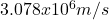Explanation:

• A) receding from the earth

The wavelength went from 434.1nm to 438.6nm, there was an increase in wavelength (also knowecn as redshift due to the doppler efft), this increase is due to the fact that the source that emits the radiation (the distant galaxy) is moving away and therefore the light waves it emits are “stretched”, causing us to see a wavelength greater than the original.

• B)to calculate the relative speed we use the following formula: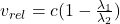where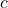is the speed of light: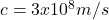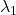is the wavelength emited by the source, and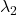is the wavelength measured on earth.

we substitute all the values and do the calculations: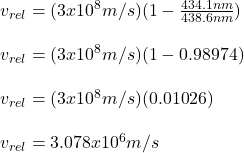the relative speed is: